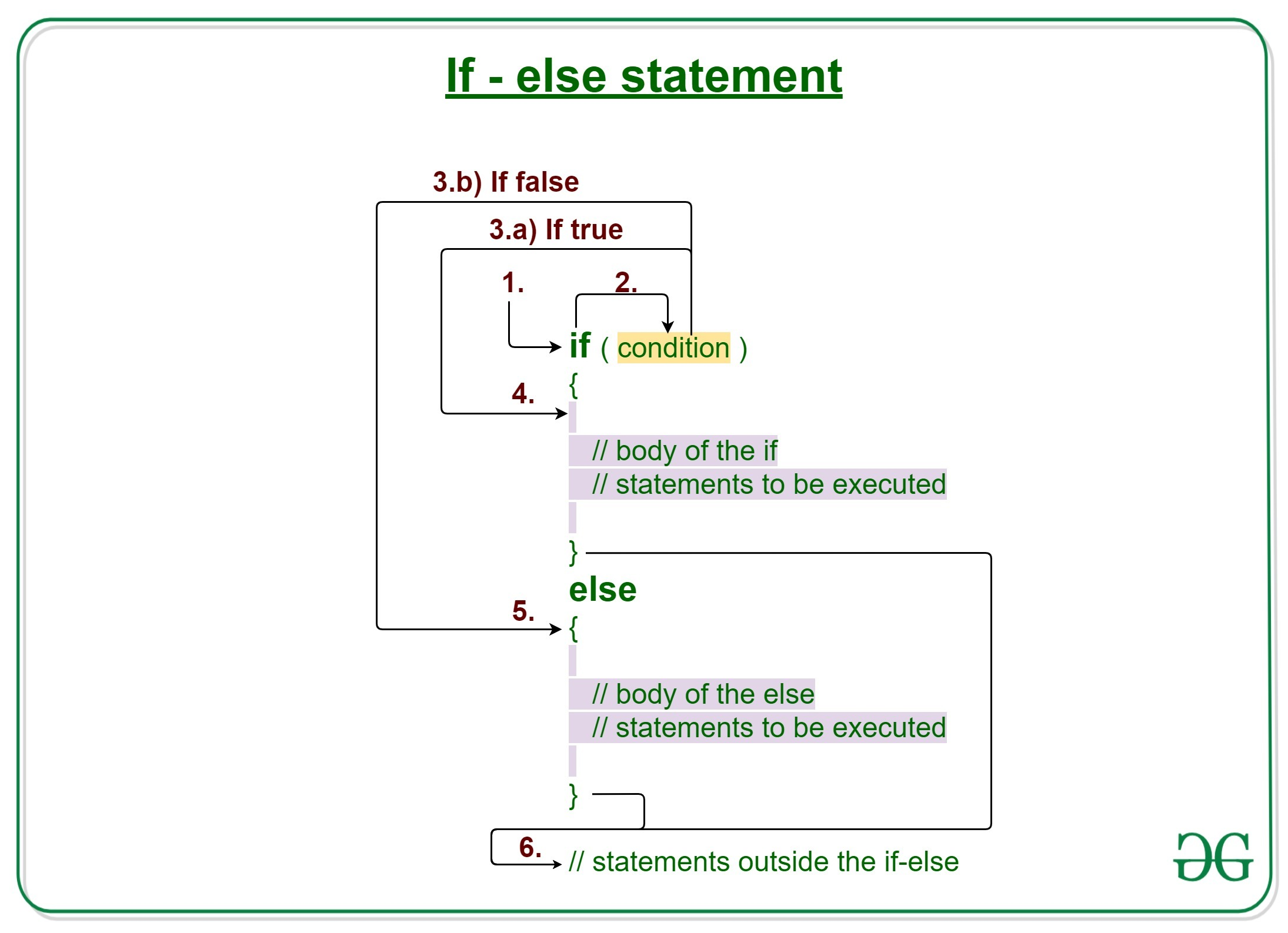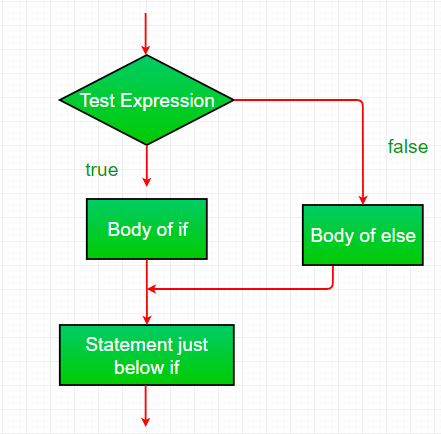Open in App
Not now

# Java if-else statement with Examples

• Difficulty Level : Easy
• Last Updated : 30 Mar, 2022

Decision Making in Java helps to write decision-driven statements and execute a particular set of code based on certain conditions.

The if statement alone tells us that if a condition is true it will execute a block of statements and if the condition is false it won’t. But what if we want to do something else if the condition is false. Here comes the else statement. We can use the else statement with the if statement to execute a block of code when the condition is false.Syntax:

```if (condition)
{
// Executes this block if
// condition is true
}
else
{
// Executes this block if
// condition is false
}```

Working of if-else statements

1. Control falls into the if block.
2. The flow jumps to Condition.
3. Condition is tested.
1. If Condition yields true, go to Step 4.
2. If Condition yields false, go to Step 5.
4. The if-block or the body inside the if is executed.
5. The else block or the body inside the else is executed.
6. Flow exits the if-else block.

Flowchart if-else:Example 1:

## Java

 `// Java program to illustrate if-else statement`   `class` `IfElseDemo {` `    ``public` `static` `void` `main(String args[])` `    ``{` `        ``int` `i = ``20``;`   `        ``if` `(i < ``15``)` `            ``System.out.println(``"i is smaller than 15"``);` `        ``else` `            ``System.out.println(``"i is greater than 15"``);`   `        ``System.out.println(``"Outside if-else block"``);` `    ``}` `}`

Output

```i is greater than 15
Outside if-else block```

Time Complexity: O(1)

Auxiliary Space: O(1)

Dry-Running Example 1:

```1. Program starts.
2. i is initialized to 20.
3. if-condition is checked. 20<15, yields false.
4. flow enters the else block.
4.a) "i is greater than 15" is printed
5. "Outside if-else block" is printed.```

Example 2:

## Java

 `// Java program to illustrate if-else statement`   `class` `IfElseDemo {` `    ``public` `static` `void` `main(String args[])` `    ``{` `        ``String str = ``"geeksforgeeks"``;`   `        ``if` `(str == ``"geeks"``)` `            ``System.out.println(``"Hello geek"``);` `        ``else` `            ``System.out.println(``"Welcome to GeeksforGeeks"``);` `    ``}` `}`

Output

`Welcome to GeeksforGeeks`

Time Complexity: O(1)

Auxiliary Space: O(1)

Related Articles:

My Personal Notes arrow_drop_up
Related Articles